# Advances on an Erdős problem on convex boundaries

Among his around 1525 papers, Paul Erdős considered On sets of distances of n points 1 as his most important contribution to discrete geometry. There, he stated:

On the boundary of every convex body, there exists a point P such that every circle with center P intersects that boundary in at most 2 points.

(Note that throughout this post all the objects considered will be in the plane). This statement can be rephrased as:

On the boundary of every planar convex body, there exists a point P such that there are no more than 2 points equidistant to P on that boundary.

However, the conjecture turned out to be false. An easy counterexample is an acute triangle, for which any point on the boundary is the center of a circle intersecting that boundary in 4 points. The following figure shows this for a particular point, but you can check the points you want at this interactive GeoGebra construction.

In fact, it turns out that any point on the boundary of a regular (2k+1)-gon is the center of a circle intersecting that boundary in 4 points. Naturally, a question arises:

Is this Erdős’s conjecture true when the upper bound 2 is replaced by 4?

Imre Bárány and Edgardo Roldán-Pensado give a negative answer to this question, constructing a convex body such that the smallest number for which Erdős’s statement holds is 6, i.e., such that for any point P on its boundary there is a circle with center P intersecting that boundary in 6 points.

The convex body they construct is a 15-gon. They consider the points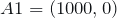,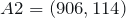,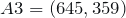, and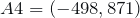, together with the points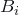and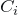, for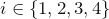, obtained by rotating the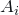‘s around the origin by an angle of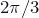and of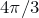, respectively. The 12-gon defined by these points looks like this

and it can be checked at this interactive GeoGebra construction. The union of the shaded regions in the figure contains the polygonal chain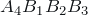and, using a technical lemma, this allows to prove that for any point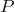in some neighborhood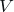of the polygonal chainthere is a circle with centerthat intersects the polygonal chain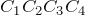in at least 6 points.

Another technical lemma, together with the fact of the angle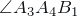being acute, implies the existence of a suitable point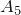. By rotational symmetry, there also exist suitable points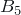and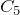, such that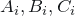for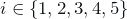form the claimed 15-gon.

In view of this construction, Bárány and Roldán-Pensado 2 conjecture that

On the boundary of every convex body, there exists a point P such that every circle with center P intersects that boundary in at most 6 points (i.e., such that there are no more than 6 points equidistant to P on that boundary).

It is interesting to note that, both the original Erdős’s conjecture and this updated one, state the existence of a universal upper bound for the number of intersections (or equidistant points), independent of the convex body considered.

Although the existence of such a universal constant remains open, Bárány and Roldán-Pensado throw some light on the problem. They prove that for every convex body there is a finite upper bound as in the statement. More formally:

For every convex body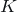, there is an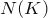being the smallest natural number for which there exists a pointon the boundary ofsuch that every circle with centerintersects the boundary ofin at mostpoints (i.e., such that there are no more thanpoints equidistant toon that boundary).

This finiteness result is actually a consequence of a stronger one. For a fixed convex body, one can define the set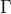of pairs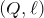in which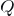is a point on the boundary of the convex body and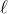is a normal to that boundary at. See the following figure.

Note that there might be several pairs for which the normals intersect at a common pointon the boundary of the convex body, like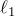and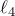in the figure. What Bárány and Roldán-Pensado prove is that there exists a pointfor which this can only happen a finite number of times. More formally:

Given a convex body, there is a pointon the boundary offor which there is a finite number of pairs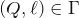such that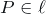and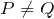.

The reason why this result implies the previous one deserves to be explained before finishing. Consider a pointon the boundary ofsuch that there are 2 points equidistant toon that boundary, call them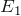and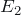. The distance betweenand these two points on the boundary is the same.

Hence, because of Rolle’s theorem, there must be a pointon the boundary, betweenand, which maximizes or minimizes the distance to. Then, for thisthere is a normalwhich contains. See the following figure.

Hence, ifis a point on the boundary offor which there are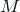pairswithand, then any circle centered atintersects the boundary in at most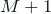points, which implies that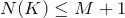. If the latter is finite, the former has to be finite as well.

As a final remark, note that this result does not imply the existence of a finite universal constant. There might exist a family of convex bodies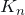with respective constants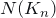such that thosetend to infinity. In that case, no constant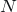would be universal, since one could always find a convexfor which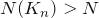, i.e., for which thatwould not be valid.

In summary, this paper by Bárány and Roldán-Pensado includes two significant advances on an Erdős problem which, despite its simple formulation, remains unsolved since 1946.

## References

1. Paul Erdős. On sets of distances of n points. The American Mathematical Monthly 53(5) (1946), 248-250. http://www.jstor.org/stable/2305092
2. Imre Bárány and Edgardo Roldán-Pensado. A Question from a Famous Paper of Erdős. Discrete and Computational Geometry. DOI: http://dx.doi.org/10.1007/s00454-013-9507-z

## Written by

• As far as I understand the conjecture says that there exists a point P, not that all the points have that special property. Therefore, I think that should not be stated that the conjecture is false because the example of the triangle.

• Dear dramey. Here comes another explanation, which I hope you find helpful.

Let us focus on the (boundary of the) acute triangle. What Erdős’s statement says is that there exists a “special” point P on the triangle, where special means having the following property:

Every circle with center P intersects the triangle in at most 2 points.”

Why is this is false? For any P you choose on the triangle, the Grinch can find a circle centered on your P and intersecting the triangle in 4 points.

Thus, the Grinch has showed that for your point P:

Not every circle with center P intersects the triangle in at most 2 points.”

because there is one intersecting in 4 points. In other words, the Grinch has showed that your point P is not “special”.

But the Grinch can do the same for any point P you choose, so there is no “special” point and hence the statement is false.

Of course, the counterexample is not mine, I just try to explain it 🙂 You can play around and emulate the Grinch with the GeoGebra interactive construction for the triangle.